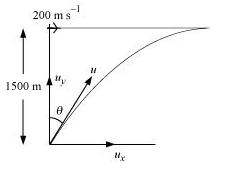## Pages

### Motion in a plane NCERT Solutions Class 11 Physics - Solved Exercise Question 4.30

Question 4.30:

A fighter plane flying horizontally at an altitude of 1.5 km with speed 720 km/h passes directly overhead an anti-aircraft gun. At what angle from the vertical should the gun be fired for the shell with muzzle speed 600 m s-1 to hit the plane ? At what minimum altitude should the pilot fly the plane to avoid being hit ? (Take g = 10 m s-2 ).

Solution:

Height of the fighter plane = 1.5 km = 1500 m
Speed of the fighter plane, v = 720 km/h = 200 m/s

Let θ be the angle with the vertical so that the shell hits the plane. The situation is shown in the given figure.Muzzle velocity of the gun, u = 600 m/s
Time taken by the shell to hit the plane = t
Horizontal distance travelled by the shell = uxt
Distance travelled by the plane = vt
The shell hits the plane. Hence, these two distances must be equal.
uxt = vt
u Sin θ = v
Sin θ = v / u
= 200 / 600 = 1/3 = 0.33
θ = Sin-1(0.33) = 19.50

In order to avoid being hit by the shell, the pilot must fly the plane at an altitude (H) higher than the maximum height achieved by the shell.
∴ H = u2Sin2 (90 - θ) / 2g
= (600)2 Cos2 θ / 2g
= 360000 X Cos2 19.5 / 2 X 10
= 16006.482 m

≈ 16 km